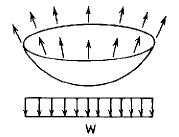Related Resources: pressure-vessel

### Uniform Loading Horizontal Projected Area Spherical Cylinder Stress and Deflection Formulas and Calculator

Spherical Cylinder Stress and Deflection Uniform loading, w force/unit area; on the horizontal projected area; tangential top edge support Equation and Calculator.

Per. Roarks Formulas for Stress and Strain for membrane stresses and deformations in thin-walled pressure vessels.

 Spherical Cylinder Stress and DeflectionUniform loading, w force/unit area; on the horizontal projected area; tangential top edge supportDeflection and Stress Uniform loading, w force/unit area; on the horizontal projected area; tangential top edge support.

For R2 / t > 10& θ ≤ 90°

Meridional StressCircumferential Hoop StressChange in height dimension yRotation of a meridian from its unloaded positionWhere used:
E = Modulus of Elasticity (lbs/in2)
v = Poisson's ratio
w = Force applied (lbs/in2)
σ1,2 = Stress, (lbs/in2)
y = Depth as indicated (in), Must be equal too or less than d
t = Wall thickness (in)
θ = Angle (deg.)
ψ = Rotation of a meridian from its unloaded position, positive when that meridional rotation represents an increase in ΔR when y or θ increases;

Reference:

Roarks Formulas for Stress and Strain, 7th Edition